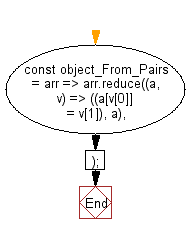# JavaScript: Create an object from the given key-value pairs

## JavaScript fundamental (ES6 Syntax): Exercise-41 with Solution

Write a JavaScript program to create an object from the given key-value pairs.

• Use Array.prototype.reduce() to create and combine key-value pairs.

Sample Solution:

JavaScript Code:

``````//#Source https://bit.ly/2neWfJ2
const object_From_Pairs = arr => arr.reduce((a, v) => ((a[v] = v), a), {});
console.log(object_From_Pairs([['a', 1], ['b', 2]]));
console.log(object_From_Pairs([[1, 10], [2, 20], [3, 30]]));
```
```

Sample Output:

```{"a":1,"b":2}
{"1":10,"2":20,"3":30}
```

Pictorial Presentation:Flowchart:Live Demo:

See the Pen javascript-basic-exercise-1-41 by w3resource (@w3resource) on CodePen.

Improve this sample solution and post your code through Disqus

What is the difficulty level of this exercise?

Test your Programming skills with w3resource's quiz.

﻿

## JavaScript: Tips of the Day

Chunks an array into n smaller arrays

Example:

```const tips_chunkIntoN = (arr, n) => {
const size = Math.ceil(arr.length / n);
return Array.from({ length: n }, (v, i) =>
arr.slice(i * size, i * size + size)
);
}
console.log(tips_chunkIntoN([1, 2, 3, 4, 5, 6, 7,8], 4));
```

Output:

```[[1,2],[3,4],[5,6],[7,8]]
```